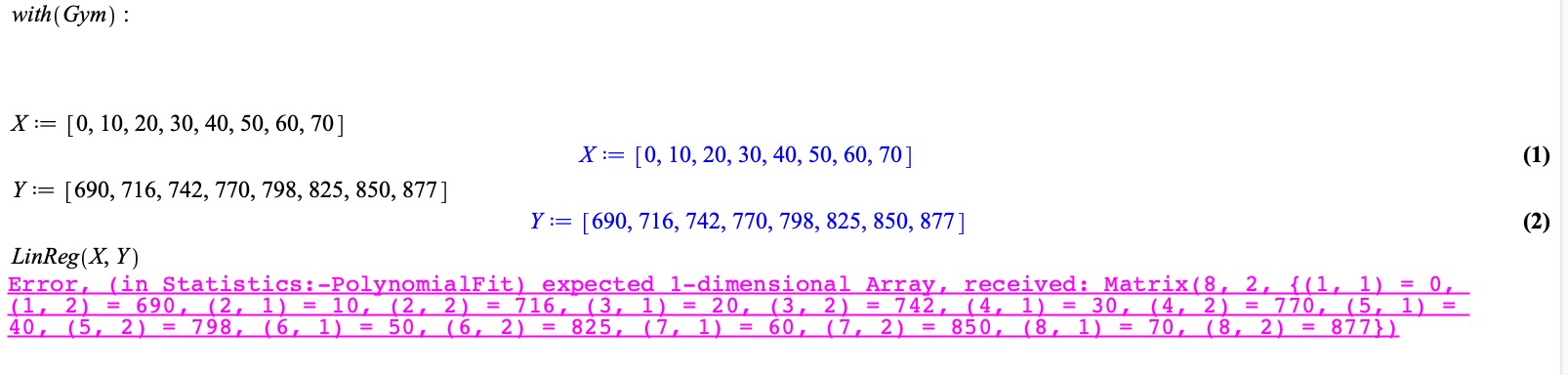## MaplePrimes Questions

Search Questions:

## Passing a string to maple using the command line...

Hello

In Section 14.5 - External Calling ..., page 489, the authors gave an example on how to use the command line in Unix to call maple with arguments. I followed the example but added a mpl-file to see if the arguments were sent to it.

Example (Linux):

`/opt/maple2020/bin/maple -c n:=4 -c 'path:=/home/eduardo/examples";' example.mpl > output.txt &`

Maple sends out the following error message: "Error, incorrect syntax in parse: '/' unexpected (near 7th character of parsed string)".

The msg is clear where the error is but I could not figure out what to do. The single quotes were supposed to take care of that, weren't they?

Many thanks.

Ed

## question on returning table from function...

I wanted to overwrite an existing variable of type table by the one returned from a call to a proc().

But it does not work, unless eval() is called on the returned result from the proc()

But if the variable that recieves the return value from the proc() was not already a table type, then it works without using eval. Why is that?

Here is an example

```restart;
foo:=proc(A::table)
return A;
end proc;

A:=table():
A["a"]:=1:
A["b"]:=2:
B:=foo(A):
whattype(B);
print(B)

table(["a" = 1, "c" = 3, "b" = 2])
```

In the above, it worked. is table now, and assigned the updated table from the proc.  But

```A:=table():
A["a"]:=1:
A["b"]:=2:
A:=foo(A): #without eval, it does not work.
whattype(A);
print(A)

symbol
A
```

I expected A to be overwritten, just like B was. To fix this, I have to change A:=foo(A): by A:=eval(foo(A)):

But why eval was not needed in the first example, and was needed for the second example?

btw, the same thing happens with Record(), not just table()

just like to understand the reasoning behind this. I expected both cases to work the same way. But it works different if the variable happend to be unassigned (like B above).

## General Tips for Numeric Integration...

Hello all,

I was hoping to get some general tips for tackling numeric integrals. As someone with little experience in the subject, I find myself overwhelemed by the many different integration methods.

Experts, what are the first steps you take when trying to find a numeric solution to an integral? How might you zero-in on a particular integration method? What about tweaking error parametrs, etc.? Is there a general framework for approaching these problems, or is it all guesswork?

Thanks!

## Express the equation ...

Hi everyone:

How can I convert the following phrase (eq) into the phrase in the photo?

eq:= -w*z^2/(2*E*MI*alpha^2)+w*H*sinh(alpha*H)*cosh(alpha*z)/(alpha^3*E*MI*cosh(alpha*H))-w*H*sinh(alpha*H)/(alpha^3*E*MI*cosh(alpha*H))+w*cosh(alpha*z)/(alpha^4*E*MI*cosh(alpha*H))-w/(alpha^4*E*MI*cosh(alpha*H))+w*H*z/(E*MI*alpha^2)-w*H*sinh(alpha*z)/(E*MI*alpha^3):

the phrase in the photo:tnx...

## A puzzle on type-specification in a proc's argumen...

I want to write a proc, say f, that takes an single argument Z, as in
f := proc(Z::?) ...
where the only acceptable Z values are pairs [x,y], where x and y are selected from the set {a,b,c,d}. The entries a,b,c,d of that set are prescribed symbolic constants.

Thus, the following are legal calls to f:
f([a,a]), f([a,b]), f([d,c])
but these are illegal:
f([a]), f([a,b,c]), f([a,x])

I don't know what to put for the "::?" in the proc's definition in order to enforce those constraints.  Any ideas?

Extra: In fact, I would prefer to ban the f([a,a]) option as well (no repeated symbols) but that's of secondary importance since it is easy to inspect and handle that within the proc's body.

## Linear regression does not work: error...